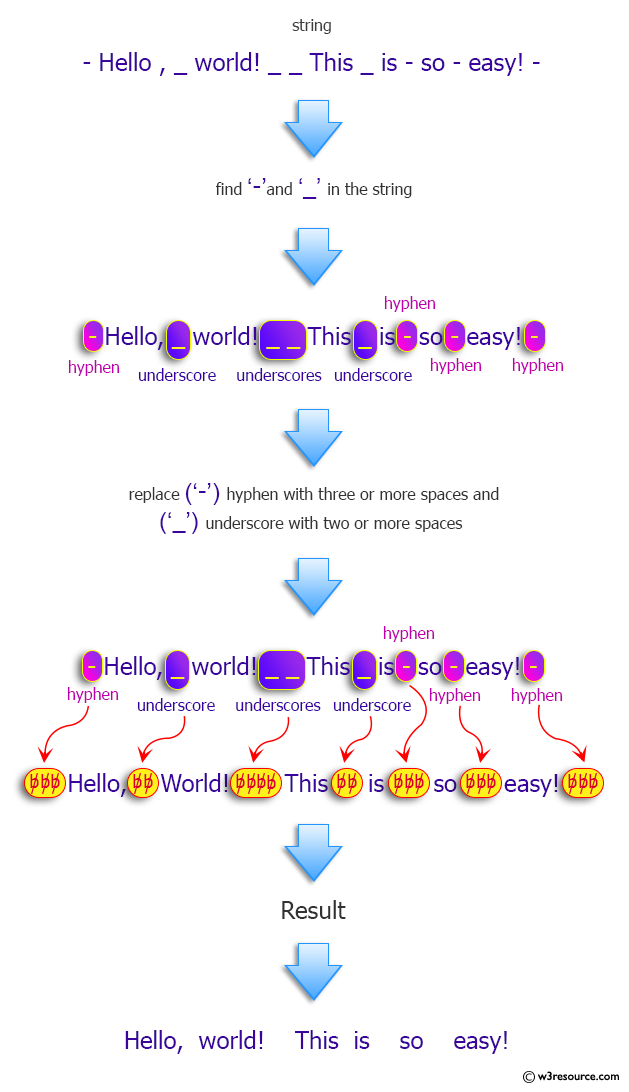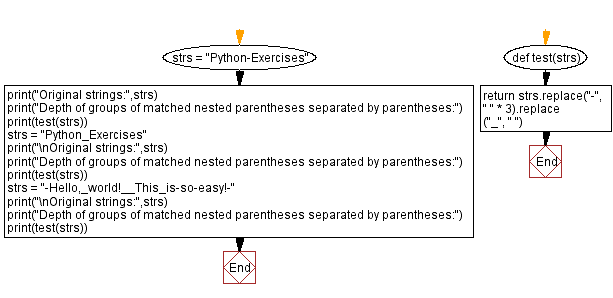﻿ Python: Expand Spaces - w3resource# Python: Expand Spaces

## Python Programming Puzzles: Exercise-99 with Solution

Write a Python program to find a string such that, when three or more spaces are compacted to a '-' and one or two spaces are replaced by underscores, leads to the target.

```Input:
Python-Exercises
Output:
Python   Exercises

Input:
Python_Exercises
Output:
Python Exercises

Input:
-Hello,_world!__This_is-so-easy!-
Output:
Hello, world!  This is   so   easy!
```

Pictorial Presentation:Sample Solution:

Python Code:

``````#License: https://bit.ly/3oLErEI

def test(strs):
return strs.replace("-", " " * 3).replace("_", " ")

strs = "Python-Exercises"
print("Original strings:",strs)
print("Depth of groups of matched nested parentheses separated by parentheses:")
print(test(strs))
strs = "Python_Exercises"
print("\nOriginal strings:",strs)
print("Depth of groups of matched nested parentheses separated by parentheses:")
print(test(strs))
strs = "-Hello,_world!__This_is-so-easy!-"
print("\nOriginal strings:",strs)
print("Depth of groups of matched nested parentheses separated by parentheses:")
print(test(strs))
``````

Sample Output:

```Original strings: Python-Exercises
Depth of groups of matched nested parentheses separated by parentheses:
Python   Exercises

Original strings: Python_Exercises
Depth of groups of matched nested parentheses separated by parentheses:
Python Exercises

Original strings: -Hello,_world!__This_is-so-easy!-
Depth of groups of matched nested parentheses separated by parentheses:
Hello, world!  This is   so   easy!
```

Flowchart:## Visualize Python code execution:

The following tool visualize what the computer is doing step-by-step as it executes the said program:

Python Code Editor :

Have another way to solve this solution? Contribute your code (and comments) through Disqus.

What is the difficulty level of this exercise?

Test your Programming skills with w3resource's quiz.

﻿

## Python: Tips of the Day

Clamps num within the inclusive range specified by the boundary values x and y:

Example:

```def tips_clamp_num(num,x,y):
return max(min(num, max(x, y)), min(x, y))
print(tips_clamp_num(2, 4, 6))
print(tips_clamp_num(1, -1, -6))
```

Output:

```4
-1
```